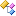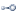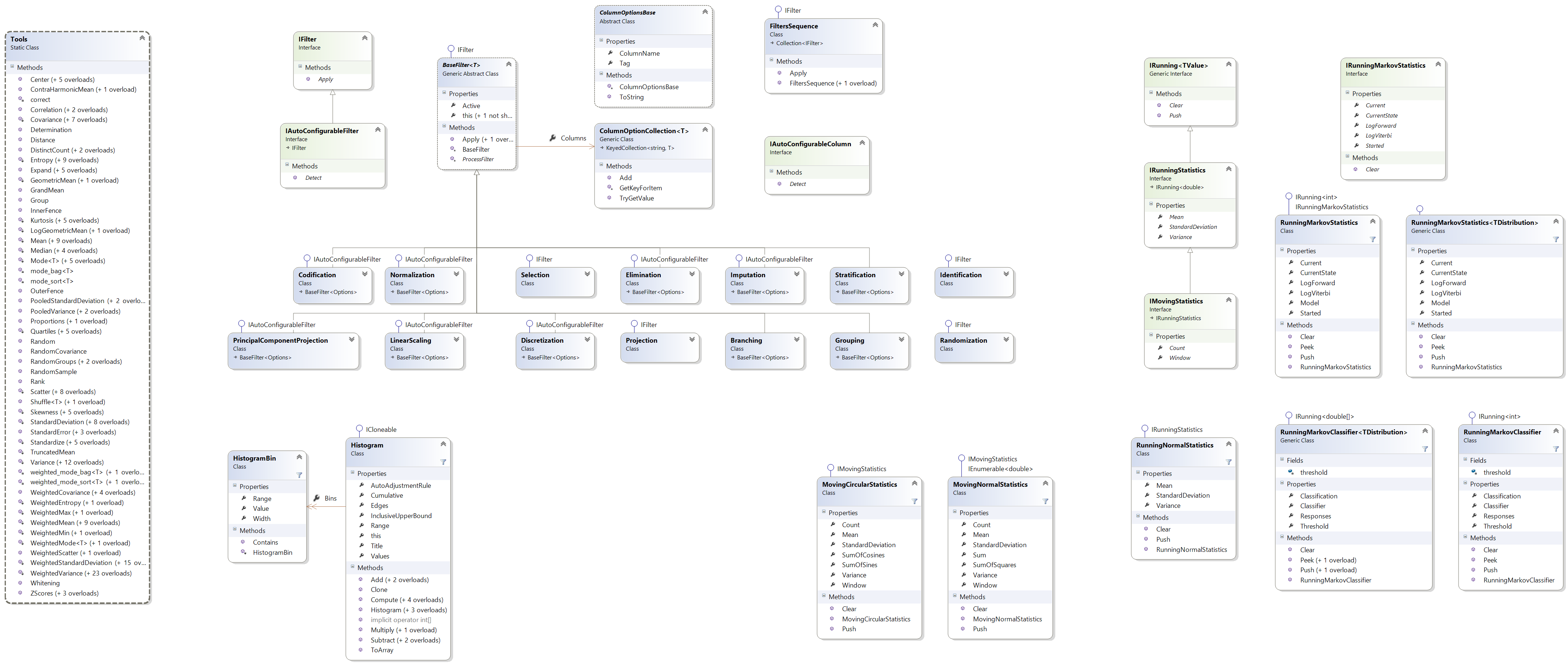﻿ Accord.Statistics.Distributions Namespace# Accord.Statistics.Distributions Namespace

Contains more than 40 statistical distributions, with support for most probability distribution measures and estimation methods.Classes
ClassDescriptionDistributionBase
Base class for statistical distribution implementations.Interfaces
InterfaceDescriptionIDistribution
Common interface for probability distributions.IDistributionTObservation
Common interface for probability distributions.IFittableTObservations
Common interface for distributions which can be estimated from data.IFittableTObservations, TOptions
Common interface for distributions which can be estimated from data.IFittableDistributionTObservations
Common interface for distributions which can be estimated from data.IFittableDistributionTObservations, TOptions
Common interface for distributions which can be estimated from data.IMixtureTDistribution
Common interface for mixture distributions.IMultivariateDistribution
Common interface for multivariate probability distributions.IMultivariateDistributionTObservation
Common interface for multivariate probability distributions.ISampleableDistributionTObservations
Common interface for sampleable distributions (i.e. distributions that allow the generation of new samples through the Generate method.IUnivariateDistribution
Common interface for univariate probability distributions.IUnivariateDistributionTObservation
Common interface for univariate probability distributions.IUnivariateFittableDistribution
Common interface for distributions which can be estimated from data.Remarks

This namespace contains a huge collection of probability distributions, ranging the from the common and simple Normal (Gaussian) and Poisson distributions to Inverse-Wishart and multivariate mixture distributions, including many specialized univariate distributions used in statistical hypothesis testing. Some of those distributions include the MannWhitneyDistribution, TDistribution, WilcoxonDistribution, KolmogorovSmirnovDistribution and many others.

For a complete list of all univariate probability distributions, check the Accord.Statistics.Distributions.Univariate namespace. For a complete list of all multivariate distributions, please see the Accord.Statistics.Distributions.Multivariate namespace.

A list of density kernels such as the Gaussian kernel and the Epanechnikov kernel are available in the Accord.Statistics.Distributions.DensityKernels namespace.

The namespace class diagram is shown below.The namespace class diagram for univariate distributions is shown below.The namespace class diagram for multivariate distributions is shown below.See Also